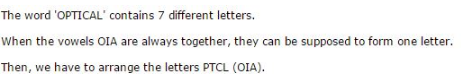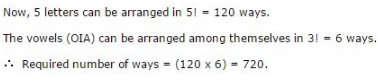# Permutation And Combination - MCQ 1

## 14 Questions MCQ Test Quantitative Aptitude for Competitive Examinations | Permutation And Combination - MCQ 1

Description
This mock test of Permutation And Combination - MCQ 1 for Quant helps you for every Quant entrance exam. This contains 14 Multiple Choice Questions for Quant Permutation And Combination - MCQ 1 (mcq) to study with solutions a complete question bank. The solved questions answers in this Permutation And Combination - MCQ 1 quiz give you a good mix of easy questions and tough questions. Quant students definitely take this Permutation And Combination - MCQ 1 exercise for a better result in the exam. You can find other Permutation And Combination - MCQ 1 extra questions, long questions & short questions for Quant on EduRev as well by searching above.
QUESTION: 1

### From a group of 7 men and 6 women, five persons are to be selected to form a committee so that at least 3 men are there on the committee. In how many ways can it be done?

Solution: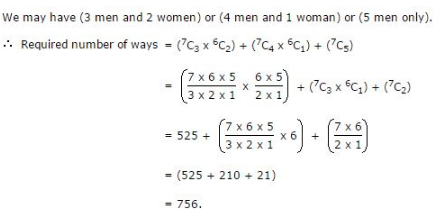QUESTION: 2

### In how many different ways can the letters of the word ‘LEADING’ be arranged in such a way that the vowels always come together?

Solution:

The word ‘LEADING’ has 7 different letters.

When the vowels EAI are always together, they can be supposed to form one letter.

Then, we have to arrange the letters LNDG (EAI).

Now, 5 (4 + 1 = 5) letters can be arranged in 5! = 120 ways.

The vowels (EAI) can be arranged among themselves in 3! = 6 ways.

∴ Required number of ways = (120 x 6) = 720.

QUESTION: 3

### In how many different ways can the letters of the word ‘CORPORATION’ be arranged so that the vowels always come together?

Solution: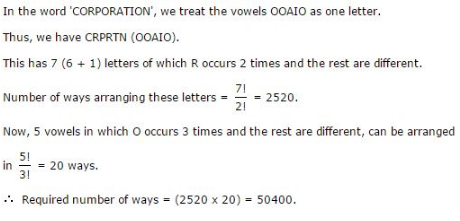QUESTION: 4

Out of 7 consonants and 4 vowels, how many words of 3 consonants and 2 vowels can be formed?

Solution: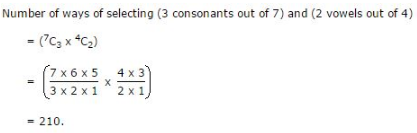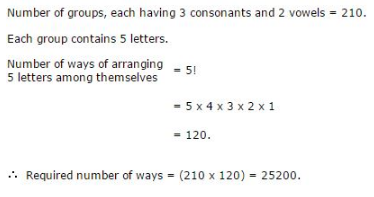QUESTION: 5

In how many ways can the letters of the word ‘LEADER’ be arranged?

Solution: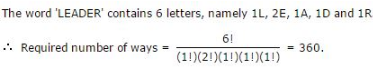QUESTION: 6

In a group of 6 boys and 4 girls, four children are to be selected. In how many different ways can they be selected such that at least one boy should be there?

Solution: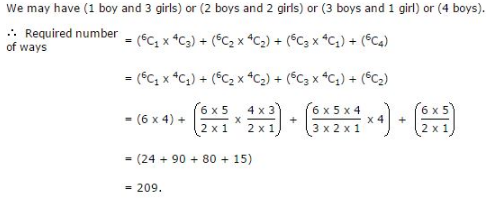QUESTION: 7

How many 3-digit numbers can be formed from the digits 2, 3, 5, 6, 7 and 9, which are divisible by 5 and none of the digits is repeated?

Solution:

Since each desired number is divisible by 5, so we must have 5 at the unit place. So, there is 1 way of doing it.

The tens place can now be filled by any of the remaining 5 digits (2, 3, 6, 7, 9). So, there are 5 ways of filling the tens place.

The hundreds place can now be filled by any of the remaining 4 digits. So, there are 4 ways of filling it.

∴ Required number of numbers = (1 x 5 x 4) = 20.

QUESTION: 8

In how many ways a committee, consisting of 5 men and 6 women can be formed from 8 men and 10 women?

Solution: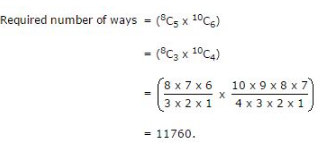QUESTION: 9

A box contains 2 white balls, 3 black balls and 4 red balls. In how many ways can 3 balls be drawn from the box, if at least one black ball is to be included in the draw?

Solution: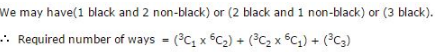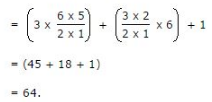QUESTION: 10

In how many different ways can the letters of the word ‘DETAIL’ be arranged in such a way that the vowels occupy only the odd positions?

Solution: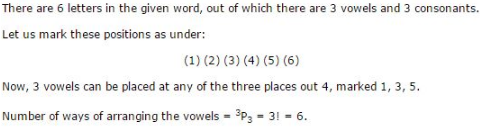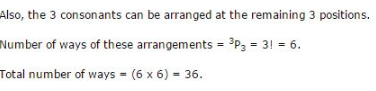QUESTION: 11

In how many ways can a group of 5 men and 2 women be made out of a total of 7 men and 3 women?

Solution: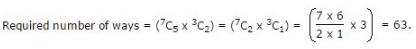QUESTION: 12

How many 4-letter words with or without meaning, can be formed out of the letters of the word, ‘LOGARITHMS’, if repetition of letters is not allowed?

Solution: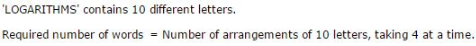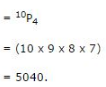QUESTION: 13

In how many different ways can the letters of the word ‘MATHEMATICS’ be arranged so that the vowels always come together?

Solution: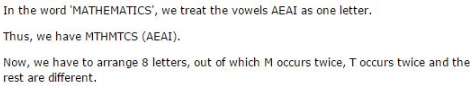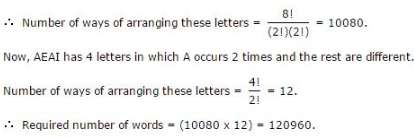QUESTION: 14

In how many different ways can the letters of the word ‘OPTICAL’ be arranged so that the vowels always come together?

Solution: